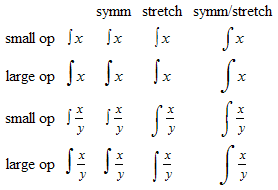prev ( testsuite > Topics > StretchyChars > Integrals > intSize2  ) next

Alternatives: (mml file)  (full5) (full) (simple) (plain) (form) (slideshow)
File:Topics/StretchyChars/Integrals/intSize2
CVS-ID:
Author:Dave Doyle, Design Science
Description:Test of integrals of various sizes under different conditions.

Sample Rendering:$\begin{array}{ccccc}& & \text{symm}& \text{stretch}& \text{symm/stretch}\\ \text{small op}& \int x& \int x& \int x& \int x\\ \text{large op}& \int x& \int x& \int x& \int x\\ \text{small op}& \int \frac{x}{y}& \int \frac{x}{y}& \int \frac{x}{y}& \int \frac{x}{y}\\ \text{large op}& \int \frac{x}{y}& \int \frac{x}{y}& \int \frac{x}{y}& \int \frac{x}{y}\end{array}$